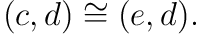### Gille-Szamuely

Posted on May 25, 2019

Here are my exercise solutions and notes for Gille and Szamuely’s Central Simple Algebras and Galois Cohomology.

1.1. Since the map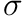is linear, for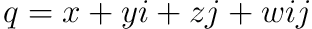we must have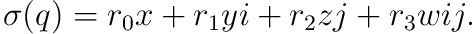To have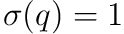we must have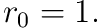Computing the product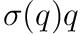and taking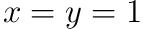,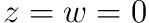we find that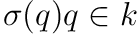only if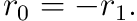Setting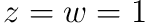and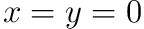we see that we must also have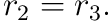Finally, setting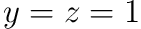and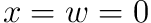we see that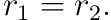Thus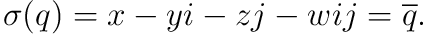1.3. Let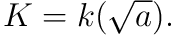Recall that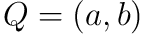for some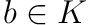by Lemma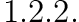Therefore let us pick the basis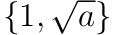for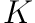and observe that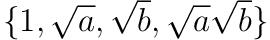is a basis for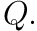Thus the quaternion norm agrees with the relative field norm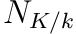for elements of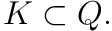1.5. Recall that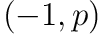splits over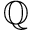if and only if the curve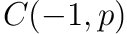defined by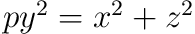has a-rational point. Observe that no-rational point on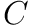has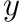-coordinate zero since such a point would have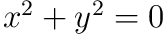for both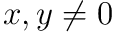, a contradiction. So all-rational points lie on the affine curve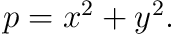By a classic result from the Gaussian integers, a prime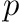may be written as the sum of two squares if and only if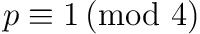(see the first section of the first chapter of Neukirch’s algebraic number theory book, for example).

Lemma. If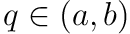such that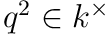then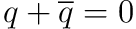or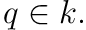Let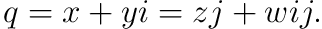Computing we find that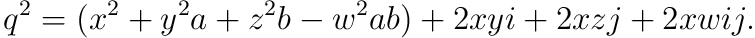Therefore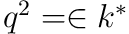if and only if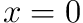or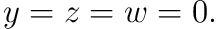1.6. Let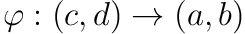be an isomorphism. Take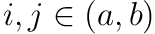such that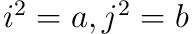, and similarly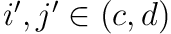such that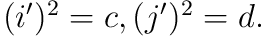Then let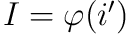and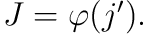Define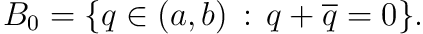Observe that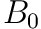is in fact a subpsace of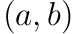of dimension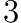and is in fact the span of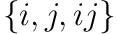(assuming that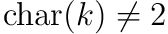we see that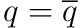implies that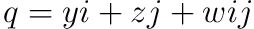).

Since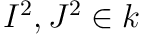, by the lemma above we have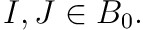Therefore we have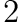-dimensional subspaces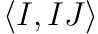and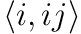in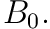Thus there is a nonzero element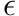in their intersection. Since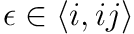we see that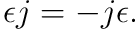Similarly, as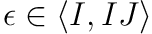we find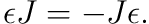Therefore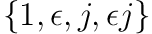is an alternate basis forand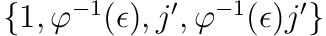is an alternate basis for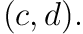Taking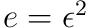we therefore have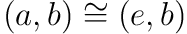and